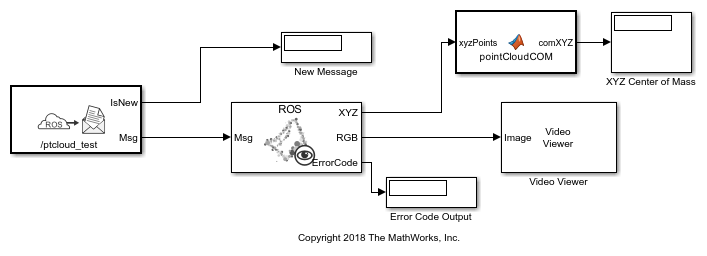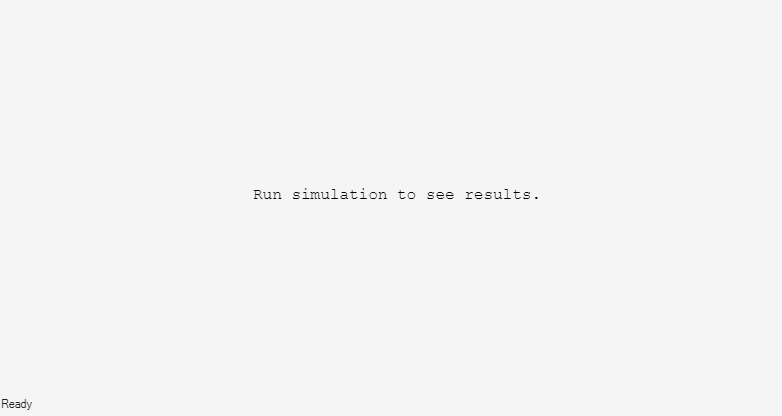Read in a point cloud message from a ROS network. Calculate the center of mass of the coordinates and display the point cloud as an image.

This example requires Computer Vision Toolbox® and Robotics System Toolbox®.

Start a ROS network.

```rosinit ```
```Initializing ROS master on http://ah-rhosea:11311/. Initializing global node /matlab_global_node_07639 with NodeURI http://ah-rhosea:51851/ ```

Load sample messages to send including a sample point cloud message, `ptcloud`. Create a publisher to send an ROS `PointCloud2` message on the `'/ptcloud_test'` topic. Specify the message type as `'sensor_msgs/PointCloud2'`. Send the point cloud message.

```exampleHelperROSLoadPCloud pub = rospublisher('/ptcloud_test','sensor_msgs/PointCloud2'); send(pub,ptcloud) ```

Open the Simulink® model for subscribing to the ROS message and reading in the point cloud from the ROS.

Ensure that the `Subscribe` block is subscribing to the `'/ptcloud_test'` topic. Under the Simulation tab, select ROS Toolbox > Variable Size Arrays and verify the `Data` array has a maximum length greater than the sample image (9,830,400 points).

The model only displays the RGB values of the point cloud as an image. The `XYZ` output is used to calculate the center of mass (mean) of the coordinates using a MATLAB Function block. All `NaN` values are ignored.

```open_system('read_point_cloud_example_model.slx') ```Run the model. The `Video Viewer` shows the sample point cloud as an image. The output center of mass is `[-0.2869 -0.0805 2.232]` for this point cloud.Stop the simulation and shut down the ROS network.

```rosshutdown ```
```Shutting down global node /matlab_global_node_07639 with NodeURI http://ah-rhosea:51851/ Shutting down ROS master on http://ah-rhosea:11311/. ```

The `pointCloudCOM` function block contains the following code for calculating the center of mass of the coordinates.

```function comXYZ = pointCloudCOM(xyzPoints) % Compute the center of mass of a point cloud based on the input NxMx3 % matrix. % Turn matrix into vectors. xPoints = reshape(xyzPoints(:,:,1),numel(xyzPoints(:,:,1)),1); yPoints = reshape(xyzPoints(:,:,2),numel(xyzPoints(:,:,2)),1); zPoints = reshape(xyzPoints(:,:,3),numel(xyzPoints(:,:,3)),1); % Calculate the mean for each set of coordinates. xMean = mean(xPoints,'omitnan'); yMean = mean(yPoints,'omitnan'); zMean = mean(zPoints,'omitnan'); comXYZ = [xMean,yMean,zMean]; end ```